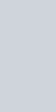• 73
•
• 2
•
•
•
•
•
75
Shares

# ELECTRICAL ENERGY AND ELECTRICAL POWER

## INTRODUCTION

[Note: some formulas text can go out of the screen, view page in landscape or desktop mode]

Electricity is the effect of the motion of electric charges that are sometimes called electrons. We know that in any electrical conductors when electrons flow there creates an electric current. This electric current is very useful for today’s world because everything almost everything is running on electricity especially the internet.Electricity has become an important part of our daily lives. We became very fed up and irritable. If electricity has gone for some minutes and hours. This has become one of our essential needs like food, water, and oxygen.

But in this article, we are not going to discuss the uses and importance of electricity. We will discuss it in some other articles.

In this article mainly we are going to discuss the physical effects created during the motion of electrons i.e electrical heat/energy and electrical power.

## ELECTRICAL ENERGY

We all know that energy is always associated with some kind of motion, in other words, we can say that energy specifies the amount of work done to move an object.

In an electric circuit, the electrons flow from one terminal to another terminal of the battery. This means something is providing energy to the electrons to flow because we know that without energy anything can’t move. So the question is who is providing energy? In an electric circuit, battery or voltage provide electrical energy.

But how do they do it? They have to only move the electrons, so in order to do this, they create the potential difference. The potential difference tends the electric charges to moves from higher potential to the lower potential. So we can say that voltage provides energy to the electrons/charges via potential difference.

So the amount of work done by the voltage on the charges/electrons in order to cause motion is called electrical energy.

When electrons move they create electric current, thus in other words, we can say that amount of energy required to generate the electric current in any circuit is called electrical energy.

When electrons move inside the conductor in a particular direction with an average velocity called drift velocity, then they move forward with several numbers of collisions with each other. These collisions of the electrons with each other cause resistance which lowers the electric current.

Due to this resistance, electric wires get hot and they radiate electrical energy in the form of heat. In any conductor, electrical energy gets converted into heat energy due to resistance, which means resistance is the factor that converts electrical energy into heat energy.

All type of energy is measured in joule. One joule of electrical energy is defined as work done needed to move one coulomb of electric charge from one terminal to another when a potential difference of one volt is applied.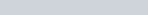### COMMERCIAL UNIT OF ELECTRICAL ENERGY

Commercial units of electrical energy are somehow big units as compared to their standard unit. This unit is used to denote the consumption of electrical energy in houses and factories.

The commercial unit of electrical energy is kWh. It is the product of power in kilos and time in hours I.e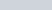. Commercial unit of electrical energy defined as it is the amount of electrical energy consumed by the electrical appliances of rated one-kilo watt in one hour.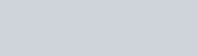If any electrical appliances consume the energy of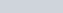then it has been called, it has consumed one unit of electricity. You must have seen a small box of electric meters on the wall of your house. This electric meter measured how much electrical energy in kWh has consumed. The cost of one unit of electricity may be 6 to 10 rupees, it may differ as per your location.

#### QUESTION

Find the value of cost electrical energy at the end of the month of April. When you run a 60W of a fan for 2hrs, 50W of TV for 3hrs, and 150W of air conditioner for 8hrs in a day. Given 1 unit of electrical energy, the cost is ₹ 10.
Solution:
Total value of electrical energy consumed in a day is-### MATHEMATICAL OPERATIONSSuppose an electric circuit has a power source of voltage V, and I is the electric current flowing through them and Q is the electric charge. Then the work done is-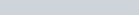We know that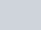So we can write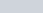So, putting the value of charge (Q) in the formula of work done, we get-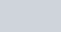We know that V = IR, put it into the above formula, we get-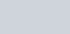We also know that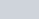, put it into the formula of work done, then we get-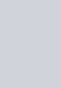## ELECTRICAL POWERPower means the rate of doing work. It can be understood as how much energy is dissipating per unit of time.

In electrical terms, electrical power is the rate at which electrical energy is transferred by an electrical circuit. In other words, we can say the rate of consumption of electrical energy.

SI unit of power is watt or joule per second and it is denoted as P. One watt of power is defined as one joule of electrical energy is dissipated in one second. Mathematically, it is given as-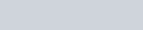### MATHEMATICAL OPERATIONS

Let’s assume a electric current having potential difference V, current I and electric charge Q. Then power is given as-We know that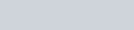We know that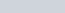, then the power is-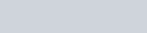We also know that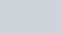, then the power is-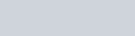## DIFFERENCE BETWEEN ELECTRICAL ENERGY AND POWER

### Electrical power

DefinitionIt is defined as the amount of work done required to move the charged particles from one terminal to another in an electrical circuit.But electrical power specifies that how much electrical energy required per second in order to move the charged particles from one terminal to another in an electrical circuit.
SI UnitIts SI unit is the jouleIts SI unit is joule per second
SymbolsIt is denoted as capital (W or E)It is denoted as P
FormulaSome formulae of electrical energy are-Some formulae of electrical power are-